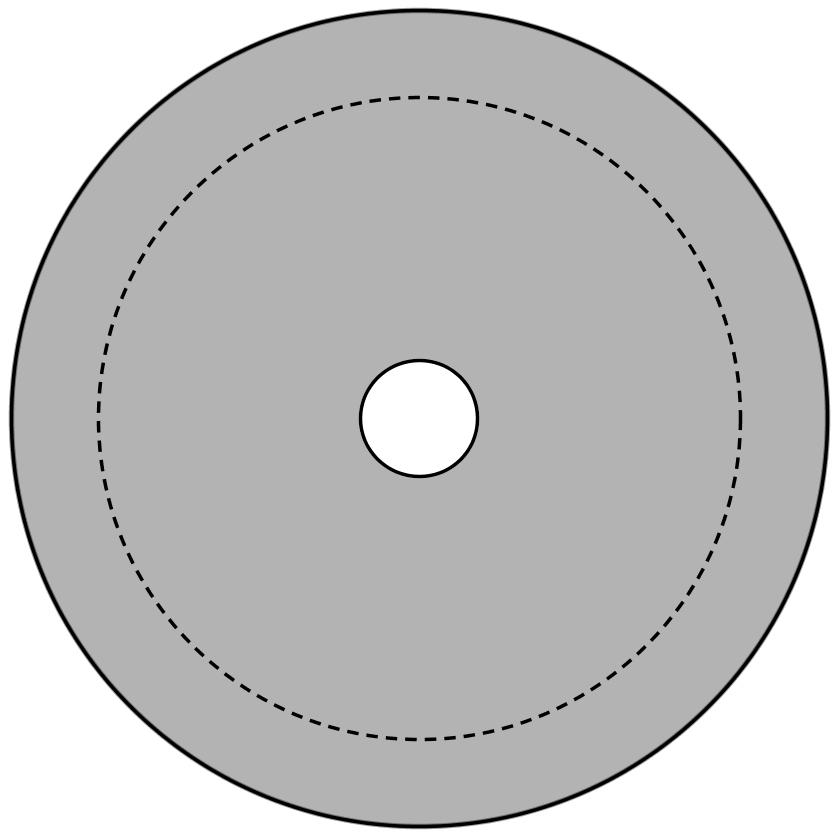#### You may also like### Dividing the Field

A farmer has a field which is the shape of a trapezium as illustrated below. To increase his profits he wishes to grow two different crops. To do this he would like to divide the field into two trapeziums each of equal area. How could he do this?### Two Circles

Draw two circles, each of radius 1 unit, so that each circle goes through the centre of the other one. What is the area of the overlap?### Star Gazing

Find the ratio of the outer shaded area to the inner area for a six pointed star and an eight pointed star.

# Half an Annulus

##### Age 14 to 16 Short Challenge Level:The shaded region in the diagram, bounded by two concentric circles (circles whose centres are at the same point), is called an annulus.

The circles have radii 2 cm and 14 cm.

The dashed circle has the same centre as the other two and divides the area of this annulus into two equal areas.

What is its radius?

This problem is taken from the UKMT Mathematical Challenges.
You can find more short problems, arranged by curriculum topic, in our short problems collection.